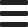# How to Create a Number Input Field in HTML

Did you know that you can create numeric input fields on your web page using the number input type thanks to HTML5? In this tutorial, I will show you how to create number input fields on your web forms.

In HTML, you can create text input fields on your web forms with the help of the <input> element. The input element is generally used for inputs that consist of a couple of words such as name, phone, email, username, password or one-line short text such as address, search query, answer to a question, etc.

To give a simple example, the following code can be used to create a text input field on your web page, simply edit your file and insert this code:

<input type="text" id="name">

The above code is used for a Name input field on a web form. Note the use of the type attribute. The input field will display like the following:

The input element is defined by its type, which may have a number of values like text, for regular text; password, for passwords; submit, for submit buttons; checkbox, for checkbox selections; radio, for radio boxes; and button for general use buttons.

The HTML5 specification, which is the official HTML recommendation now, introduced a number of new input types such as color, for color input; date, for date selection; email, for email inputs; tel for phone numbers; url for web page URLs; and number for number inputs. Some of these input types may not be supported by older browsers, in which case the input field will simply be displayed as a regular type=text field.

To create a numeric input field, i.e. a text input field that only accepts numbers as a string, we use and input element with the number type like the following:

<input type="number" id="age">

The above code is used for an Age input field and it will display like the following:

As you can see, the number input field has incremental up and down arrows on the right side. The default input value is 0, which is not displayed at the moment. Clicking the up arrow will increase the input value to 1, clicking the down arrow will decrease the value to -1. You can also enter values manually by typing into the field.

Since this is a number field, it accepts only numbers: positive and negative integers, as well as floating point numbers. You can still enter non-numeric characters such as letters or punctuation but if you try to submit the form, it will not accept and the field will display a notice asking you to enter numbers only. This notice may be different depending on the browser.

The number input field may take a number of attributes in addition to the global attributes that any HTML element can take such as the id, style and title attributes. Let's see some of them below.

## HTML Number Input Field - Disabled

You can disable a number input field using the disabled attribute like the following:

<input type="text" id="name">
<input type="number" id="age" disabled>

For example you may want to keep the age field as disabled until the person enters their name first (with the help of JavaScript).

## HTML Number Input Field - Required

To make the number input field required, use the required attribute.

<input type="number" id="size" required>

The form will not be submitted if the user does not provide a size value, since it's required.

## HTML Number Input Field - Min & Max Values

The number input field can have a range of minimum and maximum values if you wish. For example to limit the input values between 1 and 100, use the min and max attributes as shown in the example below:

<input type="number" id="quantity" min="1" max="100">

The above code will limit the quantity input between 1 and 100. You can try this on the input field below. You can also limit input length using the maxlength attribute.

## HTML Number Input Field - Step

The last attribute we will talk about is step. You can use it to specify the incremental step for the number input.

<input type="number" id="quantity" step="5">

The above code will cause the input field to increase or decrease by 5 at each click. You can test it below.

You can also use floating increment values like 0.1, 0.25, 0.5 etc. If you want your number field to accept any integer and floating point, you can use the following: